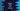# Python While and for loop : Python Tutorial 14## Python while and for loop :

Loops are used in programming language to run a piece of code again and again. In this tutorial, we will check two types of looping in python : “while” loop and “for” loop.

## Python while loop :

Take the following syntax for example :

``````while condition :
code-block()``````

i.e. if the “condition” is true , it will run the “code-block()” part . Next the condition will be checked again , it it is true , code-block() will run again. If the condition is true always, it will form a infinite loop.

``````i = 100

while i > 90 :
print "i = ",i
i = i - 1
print "while loop completed..."``````

Run this code. It will give the following output :

``````i =  100
i =  99
i =  98
i =  97
i =  96
i =  95
i =  94
i =  93
i =  92
i =  91
while loop completed…``````

Each time we are decreasing the value of i which is 100 at first. While loop is checking if i is greater that 90 or not. If i is equal or less than 90, it exists. Note that last “print” line is printed only once.

If we comment out the “i= i-1” line, it will be converted to an infinite loop. Use “Ctrl + C” to stop an infinite loop in terminal.

## while-else loop :

We can add an else block with “while” loop. It will work as “if-else”. i.e. if the condition is true, it will run the while block . If it is false , it will run “else” block.

Let’s try it with a little example :

``````i = 5

while i > 3 :
print "i = ",i
i = i - 1
else :
print "i is less than 3"
print "while loop completed..."``````

It will print :

``````i =  5
i =  4
i is less than 3
while loop completed…``````

Let’s check how “for” loop works .

## Python “for” loop :

for loop is mainly used to execute a block of code for a fixed number of times. The following example will print all numbers from 0 to 10 :

``````for x in range(0,11):
print x``````

What is this range() ? range can be defined as range( start , end , step-size ). step-size is by default 1. If we change the above program as range (0 , 11 , 2) , it will print as 0 , 2, 4 , 6 , 8, 10 . If you use range(3), then it will be from 0 to 3 i.e. 0,1 and 2.

## Nested for loops :

Nested loops means one loop inside another. Let’s check :

``````for x in range(0,3):
print "inside loop 1"
for x in range(0,2):
print "inside loop 2"``````

It will give the following output :

``````inside loop 1
inside loop 2
inside loop 2
inside loop 1
inside loop 2
inside loop 2
inside loop 1
inside loop 2
inside loop 2``````

What is happening here ? First top loop will start. it will move inside and start the inner loop since inner loop is inside first loop. So , it will continue and complete the loop 2. Same thing continues till the first loop ends .

## For with else for..else :

Similar to while, we can use else for “for” group. For the following example , what will be the output ?

``````for x in range(3):
print "x = ",x
else :
print "x is",x``````

It will have the following output :

``````x =  0
x =  1
x =  2
x is 2``````

i.e. ‘else’ block will execute after the last ‘for’ loop line . In ‘while” block we have seen above, ‘else’ block runs only if ‘while’ loop fails the condition.